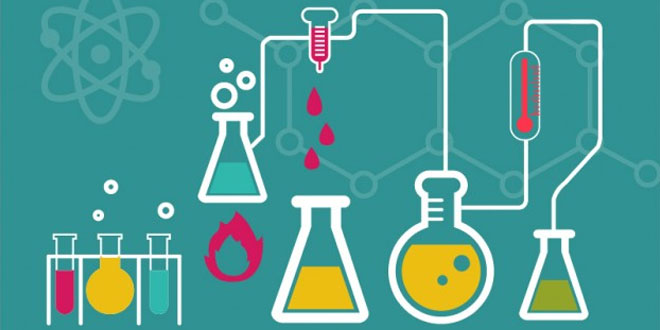Tuesday , July 5 2022# Chemical Reaction & Equations Quiz: 10 Science

## NCERT 10th Class (CBSE) Science: Chemical Reaction & Equations Quiz

#### 34 Multiple Choice Questions related to NCERT 10th Class (CBSE) Science (Chemistry) Chemical Reaction & Equations Quiz

• In a physical change, no new substance is formed while in a chemical change, a new substance is formed.
• It should be cleaned to remove the dust particles and its oxides that may be present on its upper surface, so that it comes directly in contact with air.
• The new compound formed is copper (II) chloride (CuCl2) which imparts a blue-green colour to the solution.
• Because energy in the form of heat, light or electricity is required for decomposition reactions.

# Chemical Reaction & Equations Quiz Summary

## Learning Goals:

Chemical Reaction: The transformation of chemical substance into another chemical substance is known as Chemical Reaction. For example: rusting of iron, setting of milk into curd, digestion of food, respiration, etc. In chemical reaction new substance is formed which is completely different in properties from the original substance, so in a chemical reaction chemical change takes place.

## Signs of Chemical Reaction

• Change of state of substance
• Change of colour of substance
• Evolution of heat
• Absorption of heat
• Evolution of gas
• Evolution of light
• Evolution of sound etc.

## Reactant and Product:

Reactant: Substances which take part in a chemical reaction are called reactants. Product: New substance formed after a chemical reaction is called product. Chemical Equation: Representation of chemical reaction using symbols of substances is called chemical equation. Example: A + B ⇨ C + D In this equation, A and B are called reactants and C and D are called the products. Arrow shows the direction of chemical reaction. Condition, if any, is written generally above the arrow. When hydrogen reacts with oxygen, it gives water. This reaction can be represented by following chemical equation. Hydrogen + Oxygen ⇨ Water H2 + O2 ⇨ H2O In first equation words are used and in second symbols of substances are used to write the chemical equation. For convenience, symbol of substance is used to represent chemical equations. Chemical Equation is a way to represent the chemical reaction in concise and informative way. Chemical equation can be divided into two types – Balanced Chemical Equation and Unbalanced Chemical Equation. Balanced Chemical Equation: A balanced chemical equation has number atoms of each element equal on both sides. Example: Zn + H2SO4 ⇨ ZnSO4 + H2 In this equation, numbers of zinc, hydrogen and sulphate are equal on both sides, so it is a balanced chemical equation.

According to Law of Conservation of Mass, mass can neither be created nor destroyed in a chemical reaction. To obey this law, the total mass of elements present in reactants must be equal to the total mass of elements present in products.
Unbalanced Chemical Equation: If the number of atoms of each element in reactants is not equal to the number of atoms of each element present in product, then the chemical equation is called unbalanced chemical equation. Example: Fe + H2O ⇨ Fe3O4 + H2 In this example number atoms of elements are not equal on two sides of the reaction. For example, on the left hand side only one iron atom is present, while three iron atoms are present on the right hand side. Therefore, it is an unbalanced chemical equation.

## Sources of Energy: 10th Science Chapter 14

Class: 10th Class Subject: Science Chapter: Chapter 14: Sources of Energy Quiz: – Questions MCQs: …